Home » An Introductory Guide to Regularized Greedy Forests (RGF) with a case study in Python

# An Introductory Guide to Regularized Greedy Forests (RGF) with a case study in Python

## Introduction

As a data scientist participating in multiple machine learning competition, I am always on the lookout for “not-yet-popular” algorithms. The way I define them is that these algorithms by themselves may not end up becoming a competition winner. But they bring different way for doing predictions on table.

Why I am interested in these algorithms? The key is to read “by themselves” in the statement above. These algorithms can be used in ensemble models to get extra edge over mostly popular gradient boosting algorithms (XGBoost, LightGBM etc.).

This article talks about one such algorithm called Regularized Greedy Forests (RGF). It performs comparable (if not better) to boosting algorithms for large number of datasets. They produce less correlated predictions and do well in ensemble with other tree boosting models.

In order to get the most out of this article, you should know the basics of gradient boosting and basic decision tree terminology.

• Weight Optimization
• Regularization
• Tree Size
• Model Size
2. Implementation in Python

In Boosting algorithms, each classifier/regressor is trained on data, taking into account the previous classifiers’/regressors’ success. After each training step, the weights are redistributed. Mis-classified data increases its weights to emphasize the most difficult cases. In this way, subsequent learners will focus on them during their training.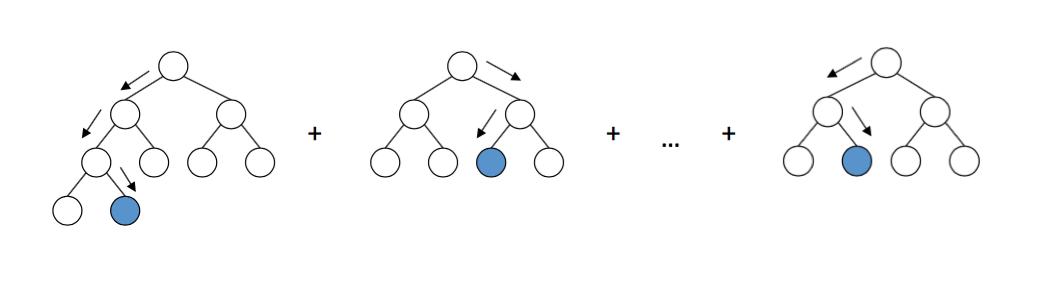However, the boosting methods simply treat the decision tree base learner as a black box and it does not take advantage of the tree structure itself.  In a sense, boosting does a partial corrective step to the model at each iteration.

In contrast, RGF performs 2 steps:

1. Finds the one step structural change to the current forest to obtain the new forest that minimises the loss function (e.g. Least squares or logloss)
2. Adjusts the leaf weights for the entire forest to minimize the loss function

Search for the optimum structure change:

1. For computational efficiency, only 2 types of operations are performed in the search strategy:
• to split an existing leaf node, and
• to start a new tree (i.e., add a new stump to the forest).
2. Search is done with the weights of all the existing leaf nodes fixed, by repeatedly evaluating the maximum loss reduction of all the possible structure changes.
3. It is expensive to search the entire forest (and that is often the case with practical applications). Hence, the search is limited to the most recently-created ‘t’ trees with the default choice of t = 1.

Let me explain this with an example.

Figure 3 shows that at the same stage as Figure 2, we may either consider splitting one of the leaf nodes marked with symbol X or grow a new tree T4 (split T4 ’s root).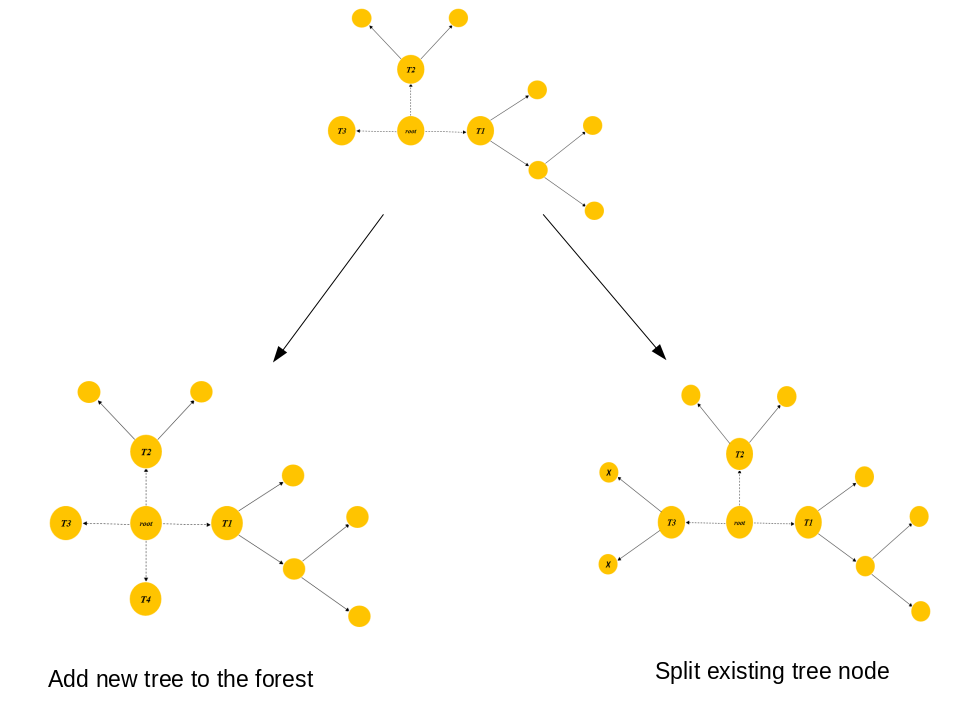Weight Optimization

Weights for each of the nodes are also optimized in order to minimize the loss function further:

1. The loss function and the interval of weight optimization can be specified by parameters. Correcting the weights every time 100 (k=100) new leaf nodes are added works well, so this is taken as a default parameter when a RGF model is trained.
2. If ‘k’ is extremely large, it would be similar to doing a single weight update at the end; if ‘k’ is extremely small (e.g., k = 1), it would really slow down the training.

Regularization

Explicit regularization to the loss function is essential for this algorithm as it overfits really quickly. It is possible to have different L2 regularization parameters for the process of growing a forest and the process of weight correction.

There are three methods of regularization:

• One is L2 regularization on leaf-only models in which the regularization penalty term G(F) is: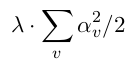• The other two are called min-penalty regularizers. Their definition of the regularization penalty term over each tree is in the form of: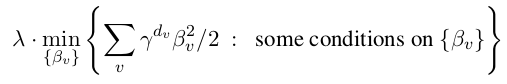A larger γ > 1 penalizes deeper nodes (corresponding to more complex functions) more severely. The degree of regularization may be adjusted through  λ or γ hyperparameters.
• Optionally, it is possible to have different L2 regularization parameters for the process of growing a forest and the process of weight correction

Tree Size

RGF does not require the tree size parameter (e.g., number of trees, max depth) needed in gradient boosted decision trees. With RGF, the size of each tree is automatically determined as a result of minimizing the regularized loss. What we do declare, is the maximum number of leaves in the forest and regularization parameters (L1 and L2).

Model Size

Since RGF performs fully corrective steps on the model/forest, it can train a simpler model as compared to boosting algorithms which require a small learning rate/shrinkage and large number of estimators to produce good results.

## Implementation in Python

Original RGF implementation for binary classification and regression was done in C++ by authors of the original research paper – Rie Johnson and Tong Zhang. The most popular wrapper of the same implementation for Python developed by fukatani even supports multiclass classification (using ‘one vs. all’ technique). Much of the implementation is based on RGF wrapper by MLWave.

### Hyperparameters

Let’s talk about the most important parameters that would affect the accuracy of the model, or speed of training, or both:

• max_leaf: Training will be terminated when the number of leaf nodes in the forest reaches this value. It should be large enough so that a good model can be obtained at some point of training, whereas a smaller value makes training time shorter.
• loss: Loss function
• LS: square loss (p-y)^2/2,
• Expo: exponential loss exp(-py)
• Log: logistic loss log(1+exp(-py))
• algorithm:
• RGF: RGF with L2 regularization on leaf-only models
• RGF Opt: RGF with min-penalty regularization
• RGF Sib: RGF with min-penalty regularization with the sum-to-zero sibling constraints
• reg_depth: Must be smaller than 1. Meant for being used with algorithm = “RGF Opt” or “RGF Sib”. A larger value penalizes deeper nodes more severely
• l2: Used to control the degree of L2 regularization. Crucial for good performance. Appropriate values are data-dependent. Either 1, 0.1, or 0.01 often produces good results though with exponential loss (loss=Expo) and logistic loss (loss=Log), some data requires smaller values such as 1e-10 or 1e-20
• sl2: Override L2 regularization parameter λ for the process of growing the forest. That is, if specified, the weight correction process uses λ and the forest growing process uses λg . If omitted, no override takes place and λ is used throughout training. On some data, λ/100 works well
• test_interval: RGF performs fully-corrective update of weights, which updates the weights of all the leaf
nodes of all the trees, in the designated interval and at the end of training.

• For this reason, if we save the model of, for example, 250 trees, then these 250 trees can be used only for testing the additive model of 250 trees. If we stopped training when ‘k’ trees were obtained, the weights assigned to the nodes of the ‘k’ trees would be totally different from those of the first ‘k’ trees of the 500 trees.
• If test interval=500, every time 500 leaf nodes are newly added to the forest, end of training is simulated and the model is tested or saved for later testing.
• normalize: If turned on, training targets are normalized so that the average becomes zero

### Training and evaluation using python wrapper

Let us try RGF on the Big Mart Sales Prediction problem. The dataset for the same can be downloaded from this datahack link. I have imported some pre-processing steps used in this article.

```import pandas as pd
import numpy as np

train['source']='train'
test['source']='test'
data = pd.concat([train, test],ignore_index=True)

#Filter categorical variables
categorical_columns = [x for x in data.dtypes.index if data.dtypes[x]=='object']

#Exclude ID cols and source:
categorical_columns = [x for x in categorical_columns if x not in ['Item_Identifier','Outlet_Identifier','source']]

#Get the first two characters of ID:
data['Item_Type_Combined'] = data['Item_Identifier'].apply(lambda x: x[0:2])

#Rename them to more intuitive categories:
data['Item_Type_Combined'] = data['Item_Type_Combined'].map({'FD':'Food',
'NC':'Non-Consumable',
'DR':'Drinks'})

#Years
data['Outlet_Years'] = 2013 - data['Outlet_Establishment_Year']

#Change categories of low fat:
data['Item_Fat_Content'] = data['Item_Fat_Content'].replace({'LF':'Low Fat',
'reg':'Regular',
'low fat':'Low Fat'})

#Mark non-consumables as separate category in low_fat:
data.loc[data['Item_Type_Combined']=="Non-Consumable",'Item_Fat_Content'] = "Non-Edible"

#Fill missing values by a very large negative val
data.fillna(-9999,inplace = True)

#Import library:
from sklearn.preprocessing import LabelEncoder
le = LabelEncoder()

#New variable for outlet
data['Outlet'] = le.fit_transform(data['Outlet_Identifier'])
var_mod = ['Item_Fat_Content','Outlet_Location_Type','Outlet_Size','Item_Type_Combined','Outlet_Type','Outlet']
le = LabelEncoder()
for i in var_mod:
data[i] = le.fit_transform(data[i].astype(str))

train_new = train.drop(['Item_Identifier','Outlet_Identifier','Item_Outlet_Sales'],axis=1)
test_new = test.drop(['Item_Identifier','Outlet_Identifier'],axis=1)
y_all = train['Item_Outlet_Sales']```

Once we have the pre-processed the stored data, we can then import RGF using the following command:

```from rgf.sklearn import RGFRegressor
from sklearn.model_selection import GridSearchCV```

The two most important parameters to set for this is the maximum number of leaves allowed and L2 regularization. We can use a grid search to find out the parameters with the best cross validation MSE.

```parameters = {'max_leaf':[1000,1200,1300,1400,1500,1600,1700,1800,1900,2000],
'l2':[0.1,0.2,0.3],
'min_samples_leaf':[5,10]}
clf = GridSearchCV(estimator=rgf,
param_grid=parameters,
scoring='neg_mean_squared_error',
n_jobs = -1,
cv = 3)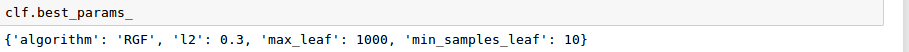```

Looks like we are trying to fit too complex a model with too many leaves. The high regularization term is high and max_leaf is low. Let us do a different grid search with lower max_leaf:

```parameters = {'max_leaf':[100,200,300,400,500,800,900,1000],
'algorithm':("RGF_Sib","RGF"),
'l2':[0.1,0.2,0.3],
'min_samples_leaf':[5,10]}```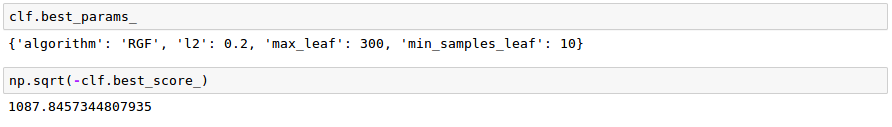Looks like these parameters are fitting the best. This scores a RMSE of 1146 on the public leaderboard.

## End Notes

RGF is yet another tree ensemble technique that sits next to gradient boosting algorithms and can be used for effectively modelling non-linear relationships. The documentation for the library used can be found at this link. There are several other parameters to try and tune with the RGF. Let me know in the comments how it works out for you.

You can also read this article on our Mobile APP•Dr.K.Vanitha Sidambaranathan says:

Excellent work Mr.Ankit; It is very helpful for us to discuss with our students…

•Ankit Choudhary says:

Thank you for the kind words.

•David says:

This is super useful! I will definitely follow your example and try it out

By saying that this algorithm “may train simpler models” what do you mean? Is it that it takes less training time or the training file weights less?

•Ankit Choudhary says:

The problem with boosting is that in order to perform well, it requires a large number of estimators with very small learning rates, while RGF constantly gets regularized at the forest level with each iteration and hence trains a model with lesser number of trees, hence the simpler model.

•Nikita says:

Thanks for the very useful and interesting article!

From Feb 10th FastRGF isn’t in alpha stage anymore and we removed FastRGF.rst document from the GitHub repo (all info is in Readme.rst document now).

•Ankit Choudhary says:

Thanks for the information.

•Ankit Choudhary says:

•Sophieandcat says: